GFG App
Open AppBrowser
Continue

Moment of inertia is the property of a body in rotational motion. It is similar to the inertia of any body in translational motion. Moment of Inertia is the property of the rotational bodies which tends to oppose the change in rotational motion. Let’s learn about the Moment of Inertia in detail in the article below.

## What is Moment of Inertia?

Moment of Inertia is the tendency of a body in rotational motion which opposes the change in its rotational motion due to external forces. Moment of Inertia behaves as angular mass and is called rotational inertia.

MOI is defined as the quantity expressed by the sum of the product of the mass of every particle with the square of its distance from the axis of rotation for any particle performing the rotational motion.

### Unit of Moment of Inertia

Moment of Inertia is a scalar quantity and the SI unit of Moment of Inertia is kg m2. The dimensional formula of the moment of inertia is, M L2

## Moment of Inertia Formula

The moment of inertia is a scalar quantity. Mathematically, the product of the square of the mass of a particle and the distance from the axis of rotation is called the moment of inertia of the particle about the axis of rotation.

General formula for finding the moment of inertia of any object is,

I = mr2

where,
m is the mass of the object’
r is the distance from the axis of rotation

Integral form of the Moment of Inertia formula is,

I = ∫dI

I =## Moment of Inertia of a System of Particles

Moment of Inertia of a system of particles is given by the formula,

I = ∑ mi ri2

where,
ri is the perpendicular distance of the ith particle from the axis
mi is the mass of ith particle

For the figure given below,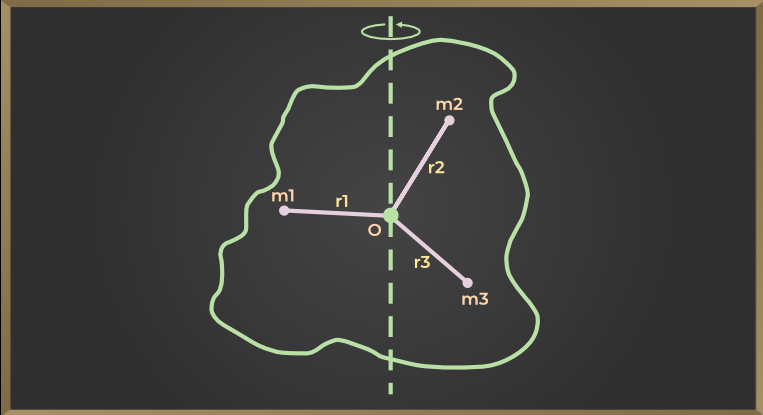Moment of inertia of first particle = m1×r12

Moment of inertia of second particle = m2×r22

Moment of inertia of third particle = m3×r3

Similarly,

Moment of inertia of nth particle = mn×rn2

Now the moment of inertia of the entire body about the axis of rotation AB will be equal to the sum of the moment of inertia of all the particles, so

I = m1×r12 + m2×r22 + m3×r3+……+mn×rn2

I = Σ mi×ri2

where,
I represent moment of inertia of the body about the axis of rotation
mi is the mass of ith particle,
ri is the radius of ith particle
Σ represents the sum.

From the equation, we can say that the moment of inertia of a body about a fixed axis, is equal to the sum of the product of the mass of each particle of that body and the square of its perpendicular distance from the fixed axis.

## Factors affecting Moment of Inertia

Moment of Inertia of any object depends on the following values:

• Shape and size of the object
• Density of the material of the object
• Axis of Rotation

## How to Calculate Moment Of Inertia?

Several ways are used to calculate the moment of inertia of any rotating object.

• For uniform objects, the moment of inertia is calculated by taking the product of its mass with the square of its distance from the axis of rotation (r2).
• For non-uniform objects, we calculate the moment of inertia by taking the sum of the product of individual point masses at each different radius for this the formula used is

I = ∑miri2

## Moment Of Inertia of Some Common Objects

Expressions for the moment of inertia for some symmetric objects along with their axis of rotation are discussed below in this table.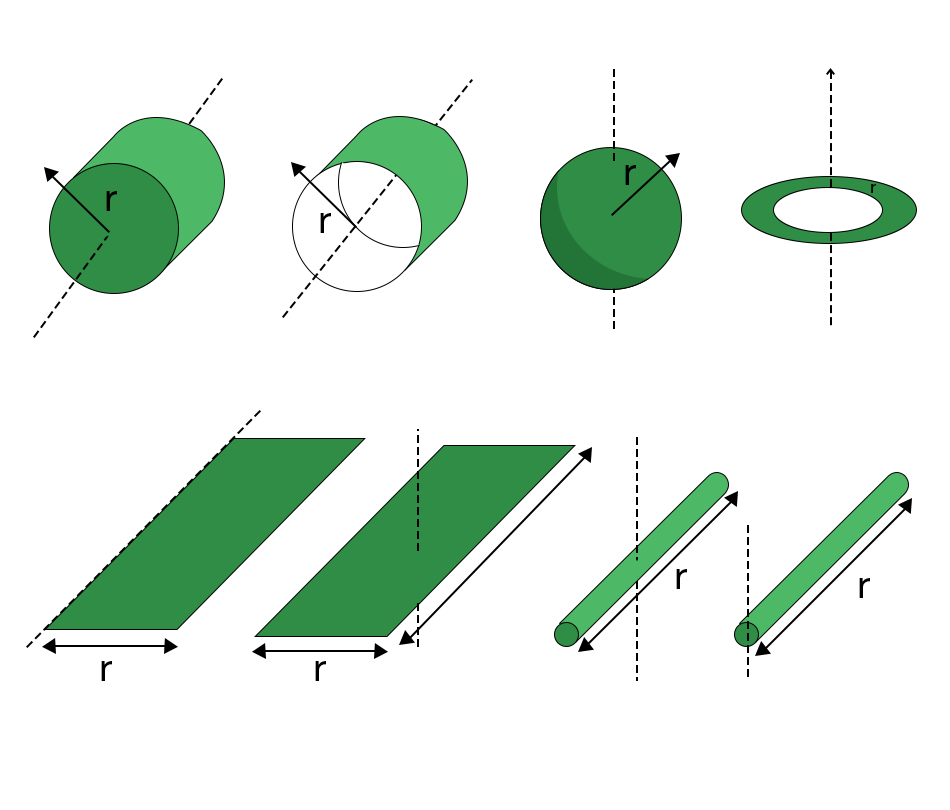The radius of gyration of a body is the perpendicular to distance from the axis of rotation to the point at which the moment of inertia obtained by taking the total mass of the body as the center is equal to the actual moment of inertia of the object. It is denoted by K.

If the mass and radius of gyration of the body are M and K respectively, then the moment of inertia of a body is

I = MK2 ……(1)

Thus, the radius of gyration of a body is that perpendicular to the axis of rotation whose square multiplied by the mass of that body gives the moment of inertia of that body about that axis.

Again by equation (1), K2 = I/M

K = √I/m

Thus, the radius of the gyration of a body about an axis is equal to the square root of the ratio of the body about that axis.

## Moment of Inertia Theorems

There are three types of theorems that are very important with respect to the Moment of Inertia:

1. Parallel Axis Theorem
2. Perpendicular Axis Theorem

## Perpendicular Axis Theorem

The sum of the moment of inertia of a body about two mutually perpendicular axes situated in the plane of a body is equal to the moment of inertia of the body about the third axis which is perpendicular to the two axes and passes through their point of intersection.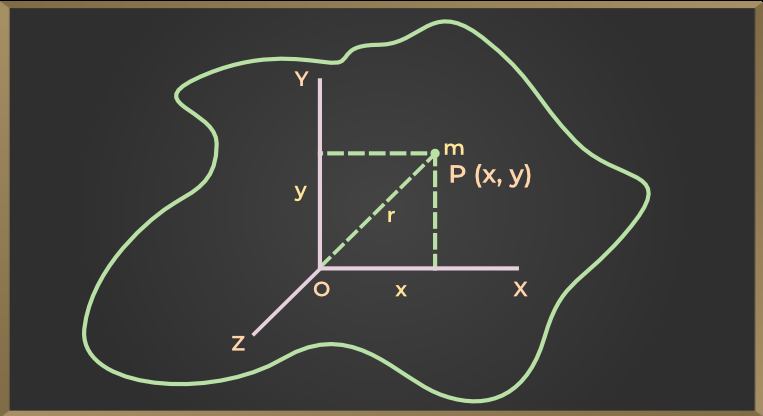In the above figure. OX and OY are two axes in the plane of the body which are perpendicular to each other. The third axis is OZ which is perpendicular to the plane of the body and passes through the point of intersection of the OX and OY axes. If Ix, Iy, and Iz are the moments of inertia of the body about the axis OX, OY, and OZ axes respectively, then according to this theorem

Ix + Iy = Iz

## Parallel Axis Theorem

According to this theorem, the moment of inertia of a body about a given axis is the sum of the moment of inertia about an axis passing through the center of mass of that body and the product of the square of the mass of the body and the perpendicular distance between the two axes.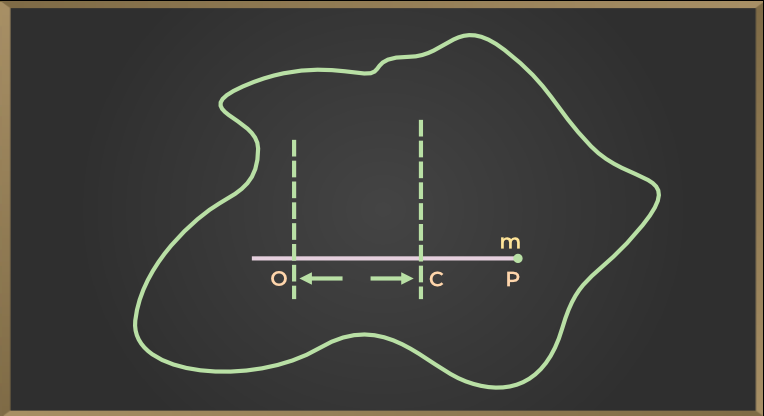Let in the above figure, we have to find the moment of inertia of IO of the body passing through the point O and about the axis perpendicular to the plane, while the moment of inertia of the body passing through the center of mass C and about an axis parallel to the given axis is IC, then according to this theorem

IO = IC + Ml2

where
M is the mass of the entire body
l is the perpendicular distance between two axes.

## Moments of Inertia for Different Objects

Moment of Inertia of different objects is discussed below in this article

### Moment of Inertia of a Rectangular Plate

If the mass of the plate is M, length l, and width b, then the moment of inertia passes through the center of gravity and about an axis perpendicular to the plane of the plate.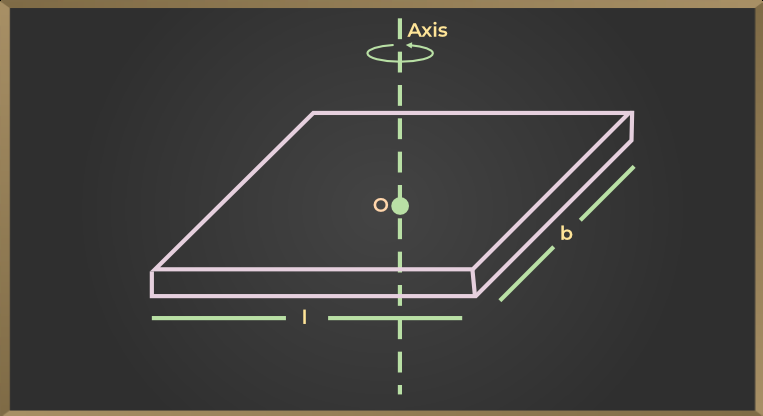I = M(l2 + b2 / 12)

### Moment of Inertia of a Disk

If the disc has a mass M and radius r, then the moment of inertia about the disc’s geometric axis is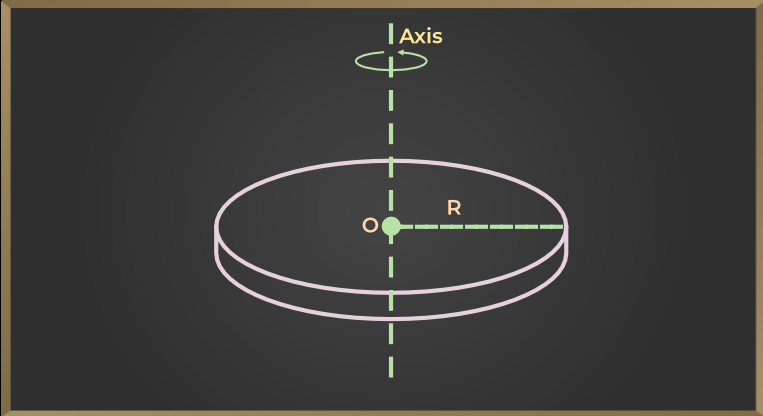I = 1/2(Mr2)

### Moment of Inertia of a Rod

If the mass of the rod is M and the length is l, then the moment of inertia about the axis perpendicular to the length of the rod and passing through its center of gravity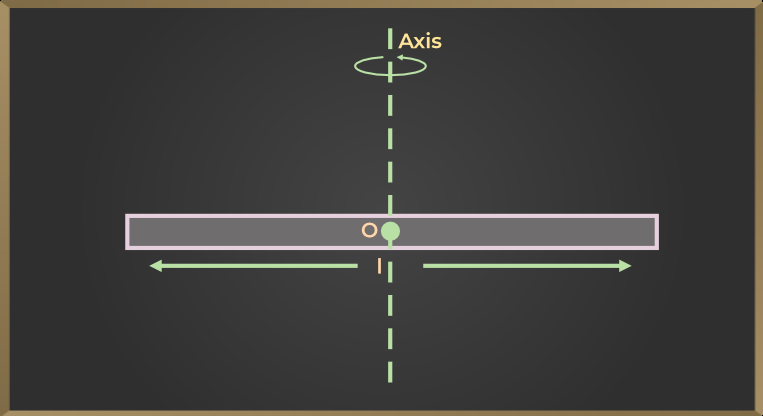I = ML2/12

### Moment of Inertia of a Circle

If the mass of the ring is M and the radius of the ring is r, then the moment of inertia about the axis passing through perpendicularly to the center of the ring is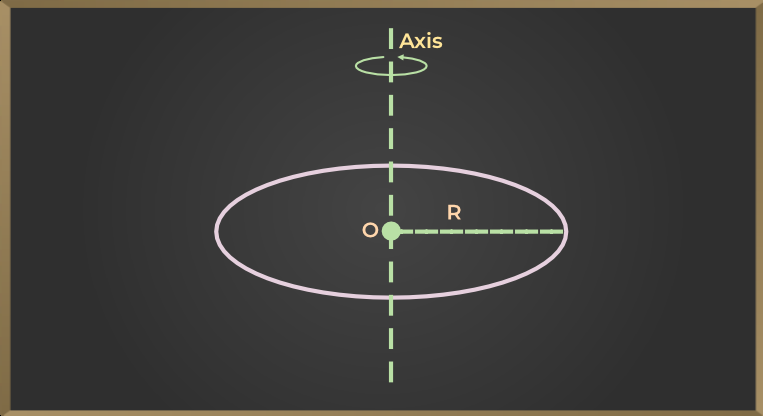I = Mr2

### Moment of Inertia of a Sphere

If a Solid Sphere has a mass of M and a radius of r, then the moment of inertia about its diameter is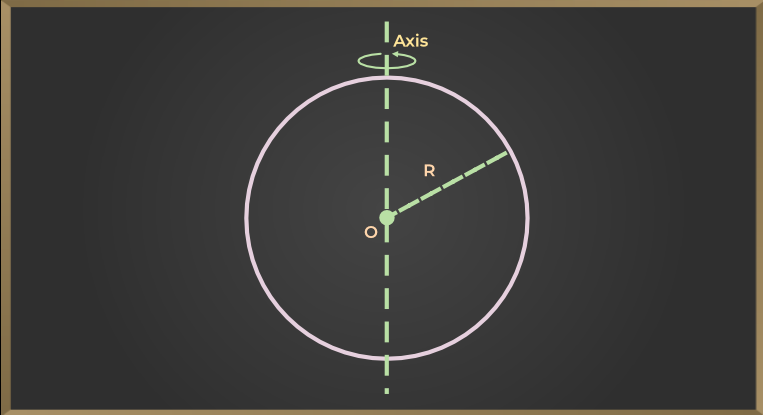I = 2/5Mr2

## Application of Moment of Inertia

MOI has various applications some of which are discussed below:

1. Due to the greater moment of inertia, the earth is rotating on its axis with the same angular velocity.
2. A small moving wheel is placed under the children’s play motor. After rubbing this wheel with the ground and leaving the motor, due to the moment of inertia of the wheel, the motor keeps running for some time.
3. Each engine consists of a large and heavy wheel attached to its shaft, with most of its mass on its circumference. Therefore, its moment of inertia is high. This wheel is called a flywheel. The torque that drives the shaft of the engine keeps on increasing. Therefore, the rotation of the shaft may not be uniform, but due to the presence of a moving wheel with more inertia, the shaft continues to rotate at an almost uniform speed.
4. In the wheel of bullock carts, rickshaws, scooters, cycles, etc., most of the mass is concentrated on its circle or rim. this hoop or routine is attached to the axis of the wheel by rigid spokes. By doing this its moment of inertia increases. Therefore, when the legs stop moving while cycling, the wheel continues to spin for some time.

Also, Check

## Solved Examples on Moments of Inertia

Example 1: A body of mass 500 g is rotating about an axis. the distance of the center of mass of the body from the axis of rotation is 1.2 m. find the moment of inertia of the body about the axis of rotation.

Solution:

Given that M = 500 g = 0.5 kg, r = 1.2 m.

Obviously, the entire mass of a body can be assumed to be placed at its center of mass. Then the moment of inertia of the body about the axis of rotation.

I = Mr2

I = 0.5 × (1.2)2

I = 0.72 kg m2

Example 2: The radius of revolution about an axis 12 cm away from the center of mass of a body of mass 1.2 kg is 13 cm. calculate the radius of revolution and moment of inertia about an axis passing through the center of mass.

Solution:

Given that, M = 1.0 kg, K = 13 cm, l = 12 cm, KCM = ?, ICM = ?

From Theorem of Parallel Axis I = ICM + Ml2

K2 = KCM2 + l2

or KCM2 = K2 – l2

KCM2 = (13)2 – (12)2 = 25

KCM = 5

Now, Moment of Inertia ICM = MKCM2

ICM = 1.0 × (0.05)2 = 2.5 × 10-3 kg m2

Example 3: A body of mass 0.1 kg is rotating about an axis. if the distance of the center of mass of the body from the axis of rotation is 0.5 m, then find the moment of inertia of the body.

Solution:

Given that, M = 0.1 kg and r = 0.5 m

so I = Mr2

I = 0.1 × (0.5)2

I = 0.025 kg m2

Example 4: The moment of inertia of the rings about an axis passing through its center perpendicular to the plane of the circular ring is 200 gm cm2. What will be the moment of inertia about its diameter?

Solution:

Moment of Inertia of a circular ring about an axis passing through another center perpendicular to its plane

MR2 = 200 gm cm2

Moment of inertia about to diameter

= 1/2 MR2

= 1/2 × 200 = 100 gm cm2

## FAQs on Moments of Inertia

Question 1: How to calculate the moment of inertia?

The basic formula for finding the moment of inertia of any uniform object is,

I = mr2

where,
m is the mass of the object’
r is the distance from the axis of rotation

Question 2: How to calculate the moment of inertia of a beam?

Moment of Inertia of a Beam along the center and axis horizontal to it is calculated using the formula,

I = ML2 / 12

Question 3: On what does the moment of inertia of a body depend?

The moment of inertia of any object depends on the factors given below:

• Mass of the Body,
• Axis of rotation
• Shape and size of the object

Question 4: What is the unit of Moment of Inertia?